# Cayley-Bacharach applications

The Cayley-Bacharach theorem in projective geometry states that if two cubics share nine points (in sufficiently general position) and a third cubic passes through eight of those points, then it also passes through the ninth. Here, ‘sufficiently general position’ means that as long as you’re not deliberately stupid with applying this theorem, it will work.

I’ve heard this referred to as a ‘super-theorem’, because its main use is to prove simpler theorems. Firstly, note this very important fact:

• The union of a degree-m and a degree-n algebraic curve is a degree-(n+m) algebraic curve.

So, for cubics, we can have either an irreducible cubic such as an elliptic curve, or the union of a conic and a line, or indeed the union of three lines. In most applications, we don’t use the full force of Cayley-Bacharach, and concentrate instead on these ‘degenerate’ cases.

### Pascal’s theorem (and converse)

Pascal’s theorem states that if we inscribe a hexagon in a conic, the extensions of opposite sides intersect at three collinear points. The hexagon can self-intersect, such as the following example:I’ve coloured each cubic in a different colour, such that the nine points are triple intersections. If we lack a single piece of information (such as a concurrency or collinearity) but know that all the other pieces of information hold, we can deduce the final piece of information through Cayley-Bacharach. For instance, the statement of Pascal’s theorem says that the red line exists, and the converse states that the red conic exists.

To determine on which branch of the cubic the point lies (either the conic or the line), we can just apply Bezout’s theorem.

### Projective dual: Brianchon’s theorem

Once we have Pascal’s theorem, we can take poles and polars about an arbitrary conic to obtain the projective dual theorem. In projective duality, we interchange lines and points, whereas conics remain conics. Concurrency of lines corresponds to collinearity of points, and so on.

The projective dual of Pascal’s theorem is called Brianchon’s theorem, which states that a hexagon circumscribed about a conic has concurrent diagonals. I’ve colour-coordinated this diagram to correspond with the diagram of Pascal’s theorem; the black lines were originally black points, and the coloured points were originally lines of that colour.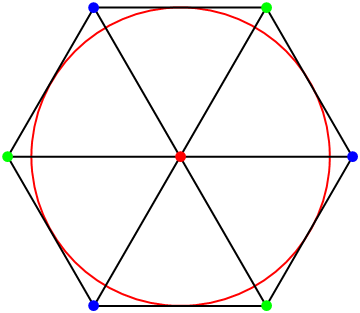We can tell that this is a projective dual of a C-B corollary rather than a C-B corollary itself, because the lines are black and the points are colourful.

Both Pascal’s theorem and Brianchon’s theorem have special ‘degenerate’ cases. If the conic in Pascal’s theorem degenerates into a pair of lines, we obtain Pappus’s theorem instead. This is also a direct corollary of Cayley-Bacharach, for obvious reasons.

In the same way that Pappus is a degenerate case of Pascal, we can view Pascal as a degenerate case of the theorem obtained by replacing the red conic and line with a single elliptic curve. This associativity law is used in elliptic curve cryptography, and more recreationally in my elliptic curve calculator. It is the most difficult part of showing that the points on an elliptic curve form a group under a geometric operation.

### Radical-axis theorem and pivot theorem

When we apply Cayley-Bacharach, we actually use the complex projective plane rather than the ordinary Euclidean plane. There are two invisible ‘circular points at infinity’, through which all circles pass. Using these, we can derive some theorems involving circles from Cayley-Bacharach. Only seven of the points are visible here; the other two are the circular points.

With three circles and three lines, there are two distinct theorems. Firstly, the pivot theorem:We also obtain the radical-axis theorem in this way:### Circle inversion

When we’re dealing with circles and lines rather than arbitrary conics, we can concentrate on the real Euclidean plane and extend it to give the Riemann sphere, or complex projective line. This is quite a sneaky trick to switch seamlessly between these two universes, but we can get away with it here. Inverting the pivot theorem yields Miquel’s theorem. This states that if we have eight points and ‘faces’ correspond to concyclic points, then five faces of a cube determine the sixth face: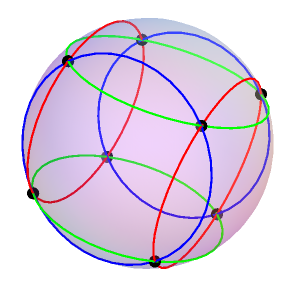The diagram above was obtained by stereographic projection, so that the cubic nature can easily be appreciated. The radical axis theorem treats the six ‘diagonal’ planes inside a cube instead of the six faces. In the very rare instances where both apply simultaneously, we get the famous orthocentric configuration.

### Quartic Cayley-Bacharach

It turns out that there is a generalisation of Miquel’s theorem to eight conics and sixteen points, but this requires the quartic analogue of Cayley-Bacharach to prove. I’ll leave this particular one as an exercise to the reader, and instead use QCB to derive some other beautiful results. There was a particularly elegant problem posted on Art of Problem Solving, the solution to which I’ll describe here.

Firstly, it’s necessary to know the statement of quartic Cayley-Bacharch. If we have 16 sufficiently-general-position points lying on two quartics, and a third quartic passes through 13 of those points, then it also passes through the other 3. In particular, if we need to prove that eight points to lie on a conic, five of them automatically do and we can get the other three through Cayley-Bacharach. Let’s have a look at the AoPS problem: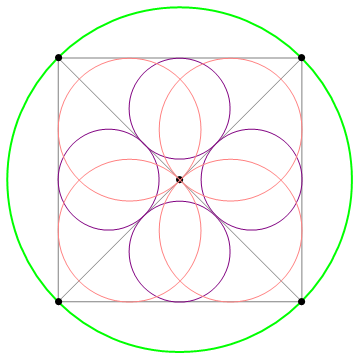We want to show that the centres of the eight pink/purple circles lie on a conic. We’ll apply the fact that they lie on the angle bisectors of the triangles. Indeed, once we’ve drawn the angle bisectors, we can forget about the grey lines and pink/purple circles completely:The red and blue angle bisectors intersect to give the eight incentres. However, they also appear to converge on the circumference of the circle to give another four points. This is not a coincidence, and can be deduced from the converse of ‘equal arcs subtend equal angles’. These sixteen points lie on the blue quartic and the red quartic, and we can also draw a green conic through five of the remaining eight points. Quartic Cayley-Bacharach then reveals that the last three points also lie on this conic: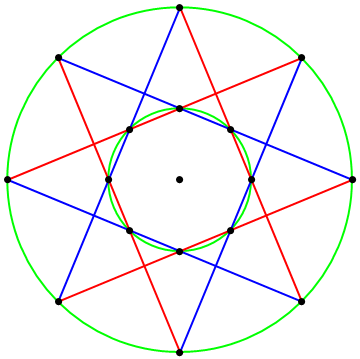This diagram is a generalisation of Pascal’s theorem, which is a corollary of generalised Cayley-Bacharach. In particular, if we have a 2n-gon inscribed in a conic, and colour alternate edges red and blue, the remaining n^2 − 2n points lie on an algebraic curve of degree n − 2. This is explored in a paper by Gabriel Katz.

### Solving an AMS problem

We’ll solve a non-trivial olympiad-style problem using as much pure projective geometry as possible. This differs completely from any ordinary way of solving the problem, although it’s somewhat neater (the standard solution has horrible Menelaus bashes and so forth).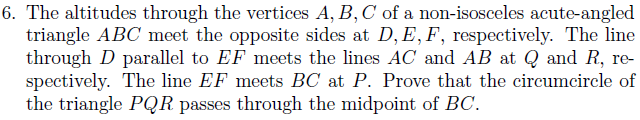Notice that Q and R are defined in quite a contrived way, so we’ll see if we can learn more about these points. It’s always good to draw a diagram in these contexts; I’ll leave it relatively uncluttered and just include F, E, B, C, Q and R (these are the only ones we’ll require at this stage).FE and QR meet at a point on the line at infinity, which is thus collinear with the two circular points. Colour the line at infinity red. We already have that the green circle exists (and has diameter BC, by Thales’ theorem), so we can conclude that BCRQ lie on a blue circle by Cayley-Bacharach. You could alternatively use an angle chase, but that’s too boring for cp4space.

The orthocentre exists; hence, (P,D;B,C) is a harmonic range. Consequently, the circle on diameter PM is the inverse of the line AD (polar of P) with respect to the circle on diameter BC (centre M). So, AD is the radical axis of circles on diameters PM and BC. This means we can just apply a degenerate case of the radical axis theorem to the points {D, Q, R, B, C, P, M} to deduce that {M, P, Q, R} are indeed concyclic.

This entry was posted in Uncategorized. Bookmark the permalink.

### 7 Responses to Cayley-Bacharach applications

1.Andrew Carlotti says:

That last paragraph was hard to follow – it would have been nice if you’d included another diagram.

2.Vinaya Kranade says:

Heello mate great blog post